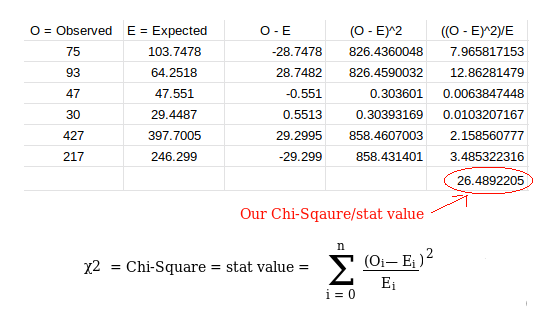# Machine Learning: How to find relationship between two categorical features.

Hello, Welcome to COT. This article explains about finding relationship between two categorical variables. We will understand the concept with an example in Python programming language. I will use 'variable', and 'feature' words interchangeably. So, don't be confused. You need some basic knowledge of Pandas library in Python to understand this article.

Categorical Variables:
Variables which take some fixed number of values. For example: If the feature name is gender, it can only have only male, and female as values. The same is applicable for a feature named weather, it can only take sunny, cloudy, and rainy etc.

If the variables are continuous, then it is easy to find relationship/correlation between them using scatter plot. But when it comes to categorical variables, we get a little bit confused. So, today I am going to explain how to use Chi-Square Test for finding if there is a relationship, or dependence between two features.

# Step by Step: How to use Chi-Square test?.

I am going to use Titanic dataset provided by kaggle. We will try find if there is a relationship between 'Embarked' (port of embarkation: C = Cherbourg, Q = Queenstown, S = Southampton), and 'Survival' features.

Step 1: Making Null Hypothesis

First, we have to make a hypothesis. We call it Null Hypothesis in the Chi-Square test of independence(no relationship). We will try to find if the hypothesis is right, or wrong using certain tests.  What's the hypothesis in our case?.

Let us call our hypothesis H0.
H0: There is no relationship between 'Embarked', and 'Survival' features.

Step 2: Get your contingency table

Contingency table:
Chi-Square test requires your data in the form of contingency table. Contingency table is nothing, but it contains frequencies of different combinations made by values of two categorical variables. You can understand it by seeing image below. I took a sample(Table no. 1) from our dataset, and Table no. 2 shows contingency table for the sample:

Table no. 3 is real contingency table for the example we are considering. Here is the code for getting contingency table for our Titanic dataset.

Embarked column contains two null values, so we have remove those two rows.

Step 3: Find Expected values.

Values stored in contingency table are called observed values. Now, we have to find expected values. To find expected values, first of all you have to find some details about contingency table like this:
Using the information in above table, we are going to find expected values table. Expected value for a certain position using above information can be calculated like following:
Scipy library in Python provides a function for all this. So, there is no need to waste your time, if you understand the math. I will do it later in this article, keep reading.

Step 4: Find Degree of Freedom(DOF), and decide Alpha.

DOF: Number of values in the table which are free to vary is called Degree of freedom. In chi-square method DOF is always:

DOF = (num_of_rows -  1)*(num_of_cols - 1)
In our case, it should be:
DOF = (3 - 1)*(2 - 1) = 2

Alpha: It is a probability value. For example, if its value is 0.05, then there are 5%(or less than 5%, you will understand later) that our H0(The Null Hypothesis) is true.

Standard value used for alpha in Chi-Square test is 0.05. So, our value for alpha is 0.05.

Step 5: Find Chi-Square value(or statistic value).

I will call this value stat for simplicity. We can find the stat value by applying Chi-Square formula on our contingency, and expected values tables. We can do it like following.We will find all the required values using Scipy in step 7.

Step 6: Find critical value of stat.

We have to find critical value of stat for our problem with the help of DOF, and alpha values in step 3. There is a standard Chi-Sqaure table for it, you just need DOF, and alpha as row, and column indexes. You can see it in the image shown below:
All these calculations can be done using just 2 Scipy functions.

Step 7: Decide whether to reject the H0 hypothesis, or not.

If the stat value is greater than critical value(5.99), then reject the hypothesis H0, otherwise you cannot reject you hypothesis. In our case, it is greater than 5.99, so we have to reject the H0. You can do it in Python like this:

1.This is a great motivational article. In fact, I am happy with your good work. They publish very supportive data, really. Continue. Continue blogging. Hope you explore your next post
360DigiTMG big data course

1.Thank you so much for your feedback. We will work hard to provide more quality, on point and easy explanation content.✌🏻

2.I would prescribe my profile is critical to me, I welcome you to talk about this point...
difference between analysis and analytics

3.Machine Learning Projects for Final Year machine learning projects for final year

Deep Learning Projects assist final year students with improving your applied Deep Learning skills rapidly while allowing you to investigate an intriguing point. Furthermore, you can include Deep Learning projects for final year into your portfolio, making it simpler to get a vocation, discover cool profession openings, and Deep Learning Projects for Final Year even arrange a more significant compensation.

Python Training in Chennai Python Training in Chennai Angular Training Project Centers in Chennai

4.Really Nice Information It's Very Helpful All courses Checkout Here.
data science course in pune

5.6.7.8.9.10.11.Good content. You write beautiful things.
sportsbet
vbet
vbet
12.13.14.15.